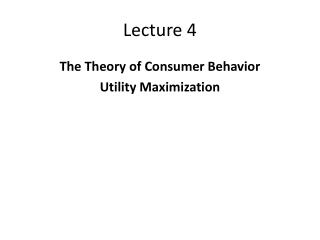DownloadDownload PresentationLecture 4

# Lecture 4

Download Presentation## Lecture 4

- - - - - - - - - - - - - - - - - - - - - - - - - - - E N D - - - - - - - - - - - - - - - - - - - - - - - - - - -
##### Presentation Transcript

1. Lecture 4 The Theory of Consumer Behavior Utility Maximization

2. Utility • Definition: Utility refers to the satisfaction derived from consuming a good or a service. • Utility is a way to describe to preference of a consumer. This implies that if I derive higher satisfaction or utility from a good then I will prefer to consume the good more. • For example: If I get more utility from good A than good B then I will prefer good A to good B.

3. Marginal Utility: As we consume more and more of a good our utility usually increases. This implies we get additional satisfaction or utility from our additional consumption. This increment to our utility is known as marginal utility.

4. As we consume more and more of a good our utility usually increases. But utility increases at a decreasing rate. This implies that the total utility will grow at a slower and slower rate. In the given example the utility increase from first unit of the good is 4. But utility increase from the second unit of good is 3. So the rate at which utility is increasing is falling. This happens because of the law of diminishing marginal utility.

5. Law of Diminishing Marginal Utility: This law states that the amount of marginal utility declines as a person consumes more and more of a good. Question: Can the marginal utility be negative? Yes.

6. Indifference Curve • Indifference Curve (IC): It shows the combination of goods or services that yields the same level of utility. That is if same level of satisfaction is derived by consuming two different bundles of goods then we can conclude that the two bundles are in the same indifference curve.

7. Example: Consider two goods X and Y. Now let us construct two bundles, bundle A= (X1, Y1) and bundle B= (X2, Y2) where X1 indicates the amount of good X and Y1 indicates the amount of good Y. Now if a consumer derives same level of satisfaction from either of the bundle then we conclude that the bundles are in the same IC. By connecting the bundles that gives the same level of utility we get the indifference curve.

8. An increase in utility is shown by a rightward shift of IC and a decrease in utility is shown by a leftward shift of IC. So in the figure IC3 shows higher utility than IC1 and IC2 shows lower utility than IC1.

9. MRS ( Marginal Rate of Substitution) • The slope of the indifference curve is known as MRS. It shows at what rate a consumer is willing to substitute one good for another good. • MRS= Slope of IC= ΔY/ΔX • So the Marginal Rate of Substitution shows that if we increase the quantity of good X ( by ΔX) then we need to decrease the quantity of good Y ( by ΔY) to keep the consumer’s utility constant.

10. Budget Constraint • Individuals have limited income or resource. That’s why we can’t consume unlimited amount of goods and services. The constraint in our income or budget is shown by budget line. • Budget Line: The budget line shows the combination of goods or services that a consumer can purchase given his limited income. • It is a downward sloping straight line. Suppose M= income X = amount of good X Px= Price of good X Y= amount of good Y Py= Price of good Y So the equation of budget line is Px*X + Py*Y= M

11. The slope of the budget line is the price ratio of the goods. Why? So the equation of budget line is Px*X + Py*Y= M Rearrange the equation Py*Y= M- Px*X Y= M/Py- (Px/Py)*X In this equation • intercept is M/Py • slope is Px/Py where Px/Py is the price ratio of the good X and Y. • So we can conclude that the slope of the budget line is the price ratio of the two goods.